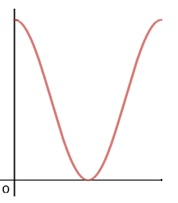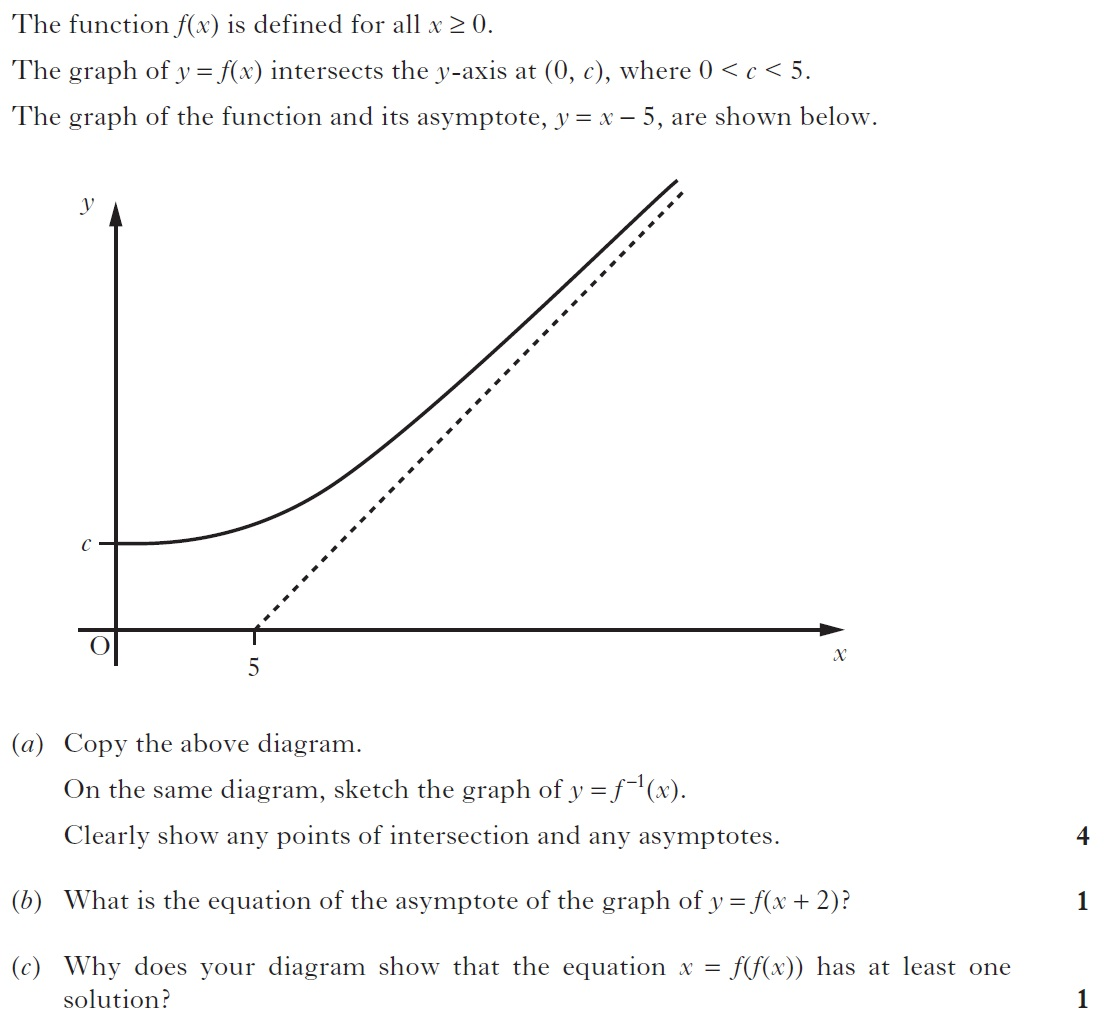# Functions – Practice questions

Q1. Consider the function  $f\left(x\right)=\sqrt{x+2}$  and  $g\left(x\right)=ax+b$ . Find  $a$  and  $b$ , if  $fog\left(1\right)=2$  and  $g\left(–1\right)=2$    .

Q2.If  $f\left(x\right)=\mathrm{sin}x$  and  $g\left(x\right)={x}^{3}$ then find the value of  $gοfο\left(gοg+g\right)$ .

Q3. If  $f\left(x\right)=\mathrm{ln}\left(\frac{x–1}{x+1}\right)$  and  $g\left(x\right)=\frac{{x}^{2}+2x}{2x+1}$ then find the value of

$gοf\left(\frac{e+1}{e–1}\right)$ .

Q4. Given  $f\left(x\right)=\frac{{25}^{x}–1}{{5}^{x}}$  , check whether  $f\left(x\right)$  is an odd function or an even function.

Q5. Check whether the function  $f\left(x\right)=\frac{x\left(1–{5}^{x}\right)}{1+{5}^{x}}$  is an odd function or an even function.

Q6. Find the value of  $f\left(\frac{1}{21}\right)+f\left(\frac{2}{21}\right)+f\left(\frac{3}{21}\right)+..............+f\left(\frac{20}{21}\right)$    if   $f\left(x\right)=\frac{{9}^{x}}{{9}^{x}+3}$  .

Q7. Find the inverse of the function  $y=\frac{{e}^{x}–{e}^{–x}}{{e}^{x}+{e}^{–x}}$  .

Q8. Find the period of the function  $y=\mathrm{sin}\left(\frac{4t+5}{3\mathrm{\pi }}\right)$  .

Q9. Find the domain of the function ${3}^{x}+{3}^{y}=3$ .

Q10. Find the value of  $a$  for which  $f\left(x\right)=\frac{ax}{x+1}$  is  $f\left(f\left(x\right)\right)=x$  .

Q11. The graph of  $f\left(x\right)$  is shown below in the domain   and range   . Sketch the graph of  $–f\left(x+2\right)+2$  .Q12.